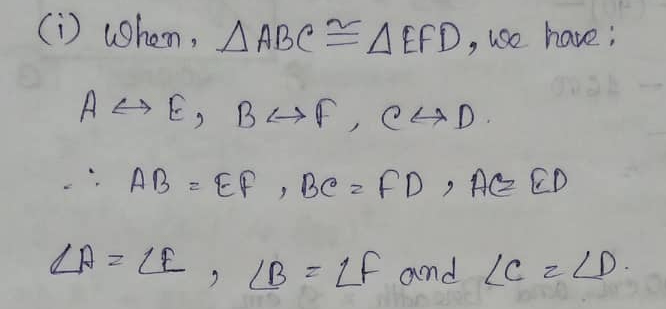# RS Aggarwal Class 7 Math Sixteenth Chapter Congruence Exercise 16 Solution

## EXERCISE 16

(1) State the correspondence between the vertices, sides and angles of the following pairs of congruent triangles.

(i) ∆ABC ≅ ∆EFD(ii) ∆CAB ≅ ∆QRP(iii) ∆XYZ ≅ ∆QRP(iv) ∆MPN ≅ ∆SQR(2) Given below are pairs of congruent triangles, State the property of congruence and name the congruent triangles in each case.(3) In Fig. (i) PL ⊥ OA and PM ⊥ OB such that PL = PM. Is ∆PLO ∆PMO?(6) In the adjoining figure, ABC is a triangle in which AD is the bisector of ∠A. If AD ⊥ BC, show that ∆ABC is isosceles.(8) In the given figure, PA ⊥ AB, QB ⊥ AB and PA = QB. Prove that ∆OAP ∆OBQ. Is OA = OB?(9) In the given figure, triangles ABC and DCB are right-angled at A and D respectively and AC = DB. Prove that ∆ABC ∆DCB.(10) In the adjoining figure, ∆ABC is an isosceles triangle in which AB = AC. If E and F be the midpoints of AC and AB respectively, prove that BE = CF.(11) In the adjoining figure, P and Q are two points on equal sides AB and AC of an isosceles triangle ABC such that AP = AQ. Prove that BQ = CP.(12) In the given figure, ∆ABC is an isosceles triangle in which AB = AC. If AB and AC are produced to D and E respectively such that BD = CE, prove that BE = CD.(13) In the adjoining figure, ∆ABC is an isosceles triangle in which AB = AC. Also, D is a point such that BD = CD. Prove that AD bisects ∠A and ∠D.(14) If two triangles have their corresponding angles equal, are they always congruent? If not, draw two triangles which are not congruent but which have their corresponding angles equal.

(15) Are two triangles congruent if two sides and an angle of one triangle are respectively equal to two sides and an angle of the other? If not then under what conditions will they be congruent?

(16) Draw ∆ABC and ∆PQR such that they are equal in area but not congruent.(17) Fill in the blanks:

(i) Two line segments are congruent if they have the same length.

(ii) Two angles are congruent if they have the same measure.

(iii) Two squares are congruent if they have the same side length.

(iv) Two circles are congruent if they have the same radius.

(v) Two rectangles are congruent if they have the same length and same breadth.

(vi) Two triangles are congruent if they have all parts equal.

(18) Which of the following statements are true and which of them false?

(i) All squares are congruent. = False

(ii) If two squares have equal areas, they are congruent. = True

(iii) If two figures have equal areas, they are congruent. = False

(iv) If two triangles are equal in area, they are congruent. = False

(v) If two sides and one angle of a triangle are equal to the corresponding two sides and angle of another triangle, the triangles are congruent. = False

(vi) If two angles and any side of a triangle are equal to the corresponding angles of another triangles then the triangles are congruent. = True

(vii) If three angles of a triangle are equal to the corresponding angles of another triangle then the triangles are congruent. = False

(viii) If the hypotenuse and an acute angle of a right triangle are equal to the hypotenuse and the corresponding acute angle of another right triangles are congruent. = True

(ix) If the hypotenuse of a triangle is equal to the hypotenuse of another right triangles are congruent. = False

(x) If two triangles are congruent then their corresponding sides and their corresponding angles are congruent. = True

Updated: May 30, 2022 — 1:38 pm